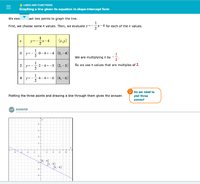# Graphing a line given its equation in slope-intercept form

#### bobmane

##### New member
I am being asked to solve for and then plot a line.
There are two versions of the problem.
One that requires doing multiplication like:
y = -2x + 5
That i know how to do.
and another that has negative fraction like
y = -1/2 +1
That one is confusing. Even when they give me the answer I am not seeing how they did the math.
Or other similar examples like y = -2/3 x 3 + 5 or y = -2/3 x 6 + 5
I attached an image of the answer they are giving:#### Dr.Peterson

##### Elite Member
and another that has negative fraction like
y = -1/2[x] +1
That one is confusing. Even when they give me the answer I am not seeing how they did the math.
Or other similar examples like y = -2/3 x 3 + 5 or y = -2/3 x 6 + 5
Are you saying that when you multiply $$\displaystyle -\frac{1}{2}\cdot2-4$$, you don't get -5, or something else?

If so, here's the work, in more detail:

$$\displaystyle -\frac{1}{2}\cdot 2-4 = \frac{-1}{2}\cdot\frac{2}{1}-4 =-1-4 = -5$$​

Does that clear it up? If not, please show your work on a problem like this, so we can see what goes wrong.

#### Jomo

##### Elite Member
You should pick multiples of 2's for x as you are multiplying x by 1/2. That 2 in the denominator WILL go into x evenly if x is a multiply of 2. Same if you have to multiply 1/3 by x. Let x be a multiple of 3.
As far as the signs go, just find the sign of the number that x is being multiplied by and the sign of x. If they are the same the results is positive, else it is negative.

#### bobmane

##### New member
Does that clear it up? If not, please show your work on a problem like this, so we can see what goes wrong.
In the other example, of Y = - 1/2 x 4 - 4
I would do:
-1/2 X 4/1 = -1 x 4 = -4 (not sure what to do with denominator) then -4 - 4 = -8 - but the answer as shown above is -6

#### Dr.Peterson

##### Elite Member
Do you not know how to multiply fractions?

-1/2 times 4 is -2, not -4. Then -2 - 4 = -6.

When you multiply fractions, you multiply the numerators (in your example, -1*4 = -4), and you multiply the denominators (2*1 = 2). The product is -4/2, which simplifies to -2. You can also simplify before multiplying, but we should stick to one thing at a time.

For advice on fractions, you might go here: https://www.mathsisfun.com/fractions_multiplication.html

#### Jomo

##### Elite Member
In the other example, of Y = - 1/2 x 4 - 4. If the x means times, then how can anyone know if when you write an x if you mean the variable x or a symbol for multiplication?
Y = (-1/2)x - 4. So x = 4
Clearly you just can't ignore the 2 in the denominator.
So you need to compute (-1/2)(4) - 4
Let's look at (-1/2)(4) first. There are to steps. One is to get the sign and another is to get the number. The sign of (-1/2) is negative and the sign of 4 is positive. So the final sign is negative (since -*- = +). Now onto finding the number, since we found the sign we can ignore the signs, (1/2)*4 can (and SHOULD) be read as half of four. Well 1/2 of 4 is 2. So we now know that (-1/2)*4 = -2
Let's finish up: (-1/2)(4) - 4 = -2 -4. You take away 2 and then take away 4 you took away 6. So (-1/2)(4) - 4 = -6.

#### bobmane

##### New member
In order to do the multiplication you must do reciprocal so in - 1/2 * 4 then you would do - 1/2 * 1/4 not * 4/1 ??

#### Subhotosh Khan

##### Super Moderator
Staff member
In order to do the multiplication you must do reciprocal so in - 1/2 * 4 then you would do - 1/2 * 1/4 not * 4/1 ??
No...No.... Double No...

You MAY use reciprocal to conduct DIVISION.

Please try to justify your work as you go along - do NOT blindly follow "must do" methods.

#### Jomo

##### Elite Member
In order to do the multiplication you must do reciprocal so in - 1/2 * 4 then you would do - 1/2 * 1/4 not * 4/1 ??
You need to think that comment though as I am 100% sure that you know that it is not true.

I am sure that you know what 6*2 equals and I am equally sure that you do not get that by computing 6*(1/2)

Here is a reason that can't work. If in multiplication you keep the 1st number and then multiply by the reciprocal of the 2nd number here is what happens.

6*2 becomes 6*(1/2). But then 6*(1/2) becomes 6*2. Then 6*2 becomes 6*(1/2) etc.... So which is correct?

Also if you multiply 6 by different numbers you should expect to get different answers. Since 2 and 1/2 are different numbers (after all you rather your boss pays you for 6 hours than for 1/2 an hour). So 6*2 can not be 6*(1/2)